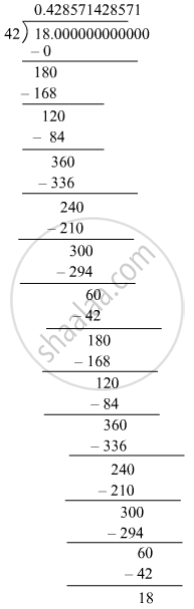SSC (English Medium) Class 8Maharashtra State Board
Share

# Write the Following Rational Number in Decimal Form. 18 42 - SSC (English Medium) Class 8 - Mathematics

ConceptConcept for Decimal Representation of Rational Numbers

#### Question

Write the following rational number in decimal form.

18/42

#### Solution

The given number is 18/42.therefore 18/42 = 0.428571428571... = 0.bar428571

"The decimal form of"  18/42  "is"  0.bar428571

Is there an error in this question or solution?

#### APPEARS IN

Balbharati Solution for Balbharati Class 8 Mathematics (2019 to Current)
Chapter 1: Rational and Irrational numbers
Practice Set 1.3 | Q: 2 | Page no. 4
Solution Write the Following Rational Number in Decimal Form. 18 42 Concept: Concept for Decimal Representation of Rational Numbers.
S